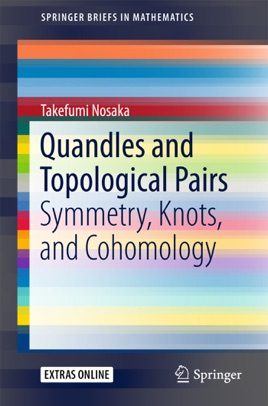• \$64.99

## Publisher Description

This book surveys quandle theory, starting from basic motivations and going on to introduce recent developments of quandles with topological applications and related topics. The book is written from topological aspects, but it illustrates how esteemed quandle theory is in mathematics, and it constitutes a crash course for studying quandles.More precisely, this work emphasizes the fresh perspective that quandle theory can be useful for the study of low-dimensional topology (e.g., knot theory) and relative objects with symmetry. The direction of research is summarized as “We shall thoroughly (re)interpret the previous studies of relative symmetry in terms of the quandle”. The perspectives contained herein can be summarized by the following topics. The first is on relative objects G/H, where G and H are groups, e.g., polyhedrons, reflection, and symmetric spaces. Next, central extensions of groups are discussed, e.g., spin structures, K2 groups, and some geometric anomalies. The third topic is a method to study relative information on a 3-dimensional manifold with a boundary, e.g., knot theory, relative cup products, and relative group cohomology.For applications in topology, it is shown that from the perspective that some existing results in topology can be recovered from some quandles, a method is provided to diagrammatically compute some “relative homology”. (Such classes since have been considered to be uncomputable and speculative). Furthermore, the book provides a perspective that unifies some previous studies of quandles.The former part of the book explains motivations for studying quandles and discusses basic properties of quandles. The latter focuses on low-dimensional topology or knot theory. Finally, problems and possibilities for future developments of quandle theory are posed.